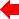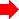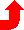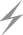Computer Aids for VLSI Design
Steven M. Rubin
Copyright © 1994

# Chapter 6: Dynamic Analysis Tools

This chapter was contributed by Robert W. Hon, Cadence Design Systems Inc.Section 1 of 8## 6.1 Introduction

Dynamic analysis of designs is the study of a circuit's behavior as a function of time. One might, for example, be interested in the logic values on a set of outputs some time t after certain logic values become present on the circuit's inputs. Or, it might be desirable to determine the expected delay from a change in an input until the output settles. In general, it is computationally impractical to determine a circuit's response to all possible inputs as a function of time; that is, it is not possible to solve in closed form the equations that represent the behavior of the circuit.

For this reason, most dynamic analysis of designs involves an empirical rather than an analytical approach to determining behavior. Simulation is the process of empirically determining a circuit's response to a particular set of inputs called a test vector. The simulation techniques that are examined here all require some model of the computational elements of the circuit and some way of handling time.

In spite of the fact that simulation is used in preference to analytical techniques for computational reasons, simulation still requires a considerable amount of computation time for circuits of only modest size. Ideally, one would prefer to simulate a design in detail. At present, this means approximating the analog-waveform behavior of the design. Simulators that operate at this level of detail are called circuit-level simulators. Circuit-level simulators give the waveform tracings for selected circuit nodes as a function of time (see Fig. 6.1). Unfortunately, circuit-level simulation is a computationally costly process and is therefore impractical for circuits of more than a few thousand transistors. Current VLSI designs consisting of a few hundred thousand transistors are well beyond the reach of circuit-level simulators.

 ``` ---------------- 0.000d+00 1.250d+00 2.500d+00 3.750d+00 5.000d+00 - - - - - - - - - - - - - - - - - - - - - - - - - - - - - 1.200d-08 4.837d-05 * . . . X 1.250d-08 4.141d-05 * . . . X 1.300d-08 3.240d-05 * . . . X 1.350d-08 2.169d-05 * . . . X 1.400d-08 1.706d-05 * . . . X 1.450d-08 1.680d-05 * . . . X 1.500d-08 1.185d-05 * . . . X 1.550d-08 -1.266d-02 * . . . = + 1.600d-08 -2.354d-02 * . . . = + 1.650d-08 -2.832d-02 * . . = . +. 1.700d-08 -1.283d-02 * . . = . +. 1.750d-08 3.128d-02 * . = . + . 1.800d-08 1.096d-01 .* . = . . + . 1.850d-08 2.285d-01 . * . = . . + . 1.900d-08 3.996d-01 . * = . . . + . 1.950d-08 6.369d-01 . =* . . . + . 2.000d-08 9.273d-01 = * . . . + . 2.050d-08 1.249d+00 = * . .+ . 2.100d-08 1.560d+00 = . * . + . 2.150d-08 1.859d+00 = . * . +. . 2.200d-08 2.146d+00 = . * . + . . 2.250d-08 2.414d+00 = . *. + . . 2.300d-08 2.661d+00 = . . * + . . 2.350d-08 2.887d+00 = . . * + . . 2.400d-08 3.093d+00 = . . *+ . . 2.450d-08 3.278d+00 = . . + * . . 2.500d-08 3.444d+00 = . . + * . . 2.550d-08 3.615d+00 . = . . + * . . 2.600d-08 3.762d+00 . = . . + * . 2.650d-08 3.885d+00 . . = . + . * . 2.700d-08 3.979d+00 . . = . + . * . 2.750d-08 4.062d+00 . . = + . * . 2.800d-08 4.132d+00 . . . += . * . 2.850d-08 4.187d+00 . . . + = . * . 2.900d-08 4.229d+00 . . + . = * . 2.950d-08 4.275d+00 . . + . . * = . 3.000d-08 4.329d+00 . . + . . * = 3.050d-08 4.381d+00 . . + . . * = 3.100d-08 4.439d+00 . . + . . * = 3.150d-08 4.498d+00 . +. . . * = 3.200d-08 4.557d+00 . + . . . * = 3.250d-08 4.614d+00 . + . . . * = 3.300d-08 4.666d+00 . + . . . * = 3.350d-08 4.714d+00 . + . . . * = 3.400d-08 4.756d+00 . + . . . * = 3.450d-08 4.794d+00 . + . . . * = 3.500d-08 4.827d+00 . + . . . * = - - - - - - - - - - - - - - - - - - - - - - - - - - - - -``` FIGURE 6.1 Circuit-level simulation output.

Since it is impossible to simulate entire complex designs at the circuit level, two alternatives exist for analyzing large designs. The first is to break the design into a number of smaller pieces and to simulate each piece at the circuit level. This technique is widely used in practice and is particularly useful when some parts of the design are used in several places (for example, an output buffer, a slice of an arithmetic-logical unit). Verification that the subpieces interact correctly can be achieved by dividing the design and simulating at the interfaces, or by other means, including hand simulation. The second method is to simulate a larger portion of the design at a coarser level of detail; for example, accepting only the logical levels 0, 1, and X (undefined) instead of full analog waveforms. As described in subsequent sections, simulators exist that view circuits as composed of switches, gates, or even more complex units. Generally, coarser approximations of behavior can be achieved in less computation time than is used for more detailed approximations, given a circuit of the same complexity.

The following sections give brief overviews of each of the main types of simulators, followed by a closer look at some issues of interest in building simulators.PreviousTable of Contents NextSteven M. Rubin Static Free Software